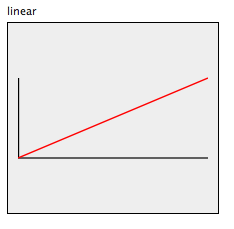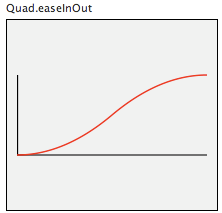Posted in: ocaml , visualization , opengl
This post is about performing advanced camera movement in OpenGL. We'll use the same Radiohead's HoC dataset we used in the previous post. Once again, the quality of the youtube video is pretty lame. You can right click here and save link as... to download a high quality version of the video (~100MB). I strongly recommend you to see the high quality video :)

Camera Instructions

Camera movement is made of Translations and/or Rotations. We want to provide our camera model with instructions of the type:
• [ Translate from to ]
• [ Rotate from to rotation_axis ]
• [ Translate ...; Rotate ... ]
As the last example shows, multiple transformations can be done at the same time (translations and rotations). The definition for a transformation type is:
type camera_op =
| Translate of Gl.point3 * Gl.point3
| Rotate of float * float * Gl.vect3
A camera instruction is a list of these operations (camera_op) and a number specifying the number of frames this transformation should take (i.e the duration of the transformation). So, for example, this instruction: ( [ Translate( (100., 100., 100.), (0., 0., 0.) ) ], 300. ) translates the camera from (100, 100, 100) to (0, 0, 0) in 300 frames, that is in 10 seconds (at 30 frames per second). Translation is done by simple interpolation. The interpolation formula for translating from A to B is something like this: A + (B - A) * delta with delta in (0, 1).

Transitions

It would be nice if camera movement, besides being linear, could also perform other advanced transitions, like the ones used in Fx.Transitions by Mootools. Some of these transitions are: Quadratic, EaseIn, EaseOut, EaseInOut, Back, Sine, etc... These effects are achieved by applying functions to the delta value, changing the way it increases or descreases its value. A possible interface for a Transition module is:
type trans = Linear | Quart
type ease = None | EaseOut | EaseIn | EaseInOut

val linear : 'a -> 'a
val quart : float -> float

val ease_in : ('a -> 'b) -> 'a -> 'b
val ease_out : (float -> float) -> float -> float
val ease_in_out : (float -> float) -> float -> float

val get_transition : trans -> float -> float
val get_ease : ease -> (float -> float) -> float -> float

val get_animation : trans -> ease -> float -> float
By using Transition.get_animation Quad EaseInOut delta we can change the timing of our animation from this:into this:Our camera instructions are then defined as:
type camera_op_list = (camera_op list) * float * (trans * ease)
For example: ( [ Translate( (100., 100., 100.), (0., 0., 0.) ) ], 300. (Quad, EaseOut))

The Camera Model

A possible interface for the camera model is:
class camera_model :
object
val mutable animations : camera_op list
val mutable time : float
val mutable total_frames : float
val mutable transition : Transition.trans * Transition.ease

method get_time : float
method step : unit
method draw : unit
method translate : Gl.point3 -> Gl.point3 -> float -> unit
method rotate : float -> float -> Gl.vect3 -> float -> unit
method set_animations :
camera_op list * float * (trans * ease) -> unit
end
The camera_model instance variables contain the destructured camera_op_list type elements: animations, total_frames and transition. We also provide individual methods for handling translations and rotations. These methods simply compute a delta value, apply the interpolation and then call GlMat.translate3 or GlMat.rotate3. The 40 line implementation looks like this:
class camera_model =
object (self)
val mutable total_frames = 0.
val mutable time = 0.
val mutable transition = (Linear, None)
val mutable animations = []

method get_time = time

method set_animations ans =
let (x, y, z) = ans in
animations <- x;
total_frames <- y;
transition <- z;
time <- 0.

method step =
if time < total_frames then
time <- time +. 1.

method translate start last delta =
let (trans, ease) = transition in
let delta_val = Transition.get_animation trans ease delta in
let (x, y, z) = start in
let (x', y', z') = last in
let DVertex(a, b, c, d) = Interpolate.cartesian
(DVertex(x, y, z, 0.))
(DVertex(x', y', z', 0.))
delta_val
in
GlMat.translate3 (a, b, c)

method rotate start last vec delta =
let (trans, ease) = transition in
let delta_val = Transition.get_animation trans ease delta in
let ang = Interpolate.cartesian_float start last delta_val in
GlMat.rotate3 ang vec

method draw =
let delta = time /. total_frames in
List.iter (fun anim ->
match anim with
| Translate(start, last) ->
self#translate start last delta
| Rotate(start, last, vec) ->
self#rotate start last vec delta ) animations
end

Timeline

Now that we have our camera model, we need a "timeline" object that can pass intructions to the camera at different stages of the animation. We define a class-less object timeline that holds a list of camera transformations to be executed at a specific frame of the animation:
let timeline =
object (self)
val mutable frame = 0.
(* Starting frame number, camera_instructions *)
val camera_timeline = [
(1.,   (* camera_instructions *));
(310., (* camera_instructions *));
(631., (* camera_instructions *) ]

method get_frame = frame

method tick =
frame <- frame +. 1.;
self#update_camera;

method update_camera =
try
let camera_anim = List.assoc frame camera_timeline in
cam#set_animations camera_anim;
with
| Not_found -> ()
end